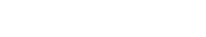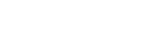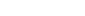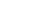# GMAT Math: Factors“How many odd factors does 210 have?”
“If n is the smallest integer such that 432 times n is the square of an integer, what is the value of y?”
“How many prime numbers are factors of 33150?”

If questions like these make you cringe, I’d like to convince you that only a few easy-to-understand concepts stand between you and doing these flawlessly.

## Idea #1: Prime Numbers

This is probably review, but just for a refresher: a prime number is any positive integer that is divisible by only 1 and itself. In other words, a prime number has only two factors: itself and 1.

Numbers that have more than two factors are called composite.

By mathematical convention, 1 is the only positive integer considered neither prime nor composite.

The first few prime numbers are:

2 3 5 7 11 13 17 19 23 29

In preparation for the GMAT, it would be good to be familiar with this list. If you verify for yourself why each number from 2 to 30 is prime or composite, it will help you remember this list.

Occasionally, the GMAT will expect you know whether a larger two-digit number, like 67, is prime.

Of course, if the number is even, it’s not prime.

If a number ends in a digit of 5, it’s divisible by five.

For divisibility by 3, a good trick to know: if the sum of the digits is divisible by three, then the number is divisible by three. Here 6 + 7 = 13, not divisible by three, so 67 is not divisible by three.

To see whether a number less than 100 is prime, all we have to do is see whether it is divisible by one of the single digit prime numbers: 2, 3, 5, or 7.

We’ve already checked 2, 3, and 5. The number 67 is not divisible by 7: 7 goes evenly into 63 and 70, not 67. That’s enough checking to establish irrevocably that 67 is prime.

## Idea #2: Prime Factorization

Every positive integer greater than 1 can be written in a unique way as a product of prime numbers; this is called its prime factorization. The prime factorization is analogous to the DNA of the number, the unique blueprint by which to construct the number. In other words, when you calculate the prime factorization of a number, you have some powerful information at your disposal.

How does one calculate the prime factorization of a number? In grade school, you may remember making “factor trees”: that’s the idea. To find the prime factorization of, for example, 48, we simply choose any two factors — say 6 and 8 — and then choose factors of those number, and then of those numbers, until we are left with nothing but primes.

Typically, once we are done, we sort the prime factors in numerical order:Once we have the prime factorization, what can we do with it? See the next two items.

## Idea #3: The Number of Factors

Suppose the GMAT asks: how many factors does 1440 have? It would be quite tedious to count them all, but there’s a fast trick once you have the prime factorization. First of all, the prime factorization of 1440 isEach prime factor has an exponent (the exponent of 5 is 1).

To find the total number of factors:
a) Find the list of exponents in the prime factorization — here {5, 2, 1}
b) Add one to each number on the list — here {6, 3, 2}
c) Multiply those together —The number 1440 has thirty-six factors, including 1 and itself.
Suppose the GMAT asked the number of odd factors of 1440. We know that odd factors cannot contain any factor of 2 at all, so basically we repeat that procedure with all the factors except the factors of 2. Here: {2, 1} –> {3, 2} –>. The number 1440 has 6 odd factors, including 1. Just for verification, the odd factors of 1440 are

{1, 3, 5, 9, 15, and 45}

This also means it has 36 – 6 = 30 even factors.

## Idea #4: GCF and LCM

GCF = greatest common factor

LCM = least common multiple.

(Note: LCM and LCD are the same thing: a least common denominator, LCD, of two number is always their LCM.)

Suppose a GMAT Math question involves finding, say, the LCM (or LCD) of 30 and 48. There’s a very straightforward procedure to find the LCM.

• Find the prime factorizations of the two numbers: 30 = 2*3*5 and 48 = 2*2*2*2*3
• Find the factors they have in common – the product of these is the GCF.  Here, the GCF = 2*3 = 6
• Express each number as the GCF*(other stuff): 30 = 6*5 and 48 = 6*8
• The LCM = GCF*(other stuff from first number)*(other stuff from the second number): LCM = 6*5*8 = 240

Let’s do one more, just for practice. Suppose, on a GMAT math problem, we need to find the LCM/LCD of 28 and 180

Step (a): 28 = 2*2*7, 180 = 2*2*3*3*5

Step (b): 28 = 2*2*7, 180 = 2*2*3*3*5; GCF = 2*2 = 4

Step (c) 28 = 4*7, 180 = 4*45

Step (d) LCM = 4*7*45 = 1260

## Practice Questions:

1) The number of boxes in a warehouse can be divided evenly into 6 equal shipments by boat or 27 equal shipments by truck. What is the smallest number of boxes that could be in the warehouse?
(A) 27
(B) 33
(C) 54
(D) 81
(E) 162

2) How many odd factors does 210 have?
(A) 3
(B) 4
(C) 5
(D) 6
(E) 8

3) If n is the smallest integer such that 432 times n is the square of an integer, what is the value of n?
(A) 2
(B) 3
(C) 6
(D) 12
(E) 24

4) How many distinct prime numbers are factors of 33150?
(A) Four
(B) Five
(C) Six
(D) Seven
(E) Eight

5) If n is a positive integer, then n(n + 1)(n – 1) is
(A) even only when n is even
(B) odd only when n is even
(C) odd only when n is odd
(D) always divisible by 3
(E) always one less than a prime number

1) C
2) E
3) B
4) B
5) D

## Explanations

1) This tells us that the number of boxes is evenly divisible by both 6 and 27; in other words, it’s a common multiple of 6 and 27. The question says: what’s the smallest value it could have? In other words, what’s the LCM of 6 and 27? (This question is one example of a real-world set-up where the question is actually asking for the LCM.)

Step (a): 6 = 2*3 27 = 3*3*3

Step (b): 6 = 2*3 27 = 3*3*3 GCF = 3

Step (c): 6 = 3*2 27 = 3*9

Step (d) LCM = 3*2*9 = 54

Thus, 54 is the LCM of 6 and 27.

For odd factors, we put aside the factor of two, and look at the other prime factors.
set of exponents = {1, 1, 1}
plus 1 to each = {2, 2, 2}
product = 2*2*2 = 8
Therefore, there are 8 odd factors of 210. In case you are curious, they are {1, 3, 5, 7, 15, 21, 35, and 105}

3) The prime factorization of a square has to have even powers of all its prime factors. If the original number has a factor, say of 7, then when it’s squared, the square will have a factor of 7^2. Another way to say that is: any positive integer all of whose prime factors have even powers must be a perfect square of some other integer. Look at the prime factorization of 432

432 = (2^4)*(3^3)

The factor of 2 already has an even power —- that’s all set. The factor of 3 currently has an odd power. If n = 3, then 432*n would have an even power of 2 and an even power of 3; therefore, it would be a perfect square. Thus, n = 3 is a choice that makes 432*n a perfect square.

33150 = 50*663 = (2*5*5)*3*221 = (2)*(3)*(5^2)*(13)*(17)

There are five distinct prime factors, {2, 3, 5, 13, and 17}

5) Notice that (n – 1) and n and (n + 1) are three consecutive integers. This question is about the product of three consecutive integers.

If n is even, then this product will be (odd)*(even)*(odd) = even

If n is odd, this this product will be (even)*(odd)*(even) = even

No matter what, the product is even. Therefore, answers (A) & (B) & (C) are all out.

Let’s look at a couple examples, to get a feel for this

3*4*5 = 60
4*5*6 = 120
5*6*7 = 210
6*7*8 = 336
7*8*9 = 504

Notice that one of the three numbers always has to be a multiple of 3: when you take any three consecutive integers, one of them is always a multiple of 3. Therefore the product will always be divisible by 3.

BTW, for answer choice E of that question, you will notice that for some trios of positives integers, adding one to the product does result in a prime, but for others, it doesn’t.

3*4*5 + 1 = 61 = prime
4*5*6 + 1 = 121 = 11^2 (not prime)
5*6*7 + 1 = 211 = prime
6*7*8 + 1 = 337 = prime
7*8*9 + 1 = 505 = 5*101 (not prime)

This is a mathematical idea far far more advanced than anything on the GMAT, but it is mathematically impossible to create an easy rule or formula that will always result in prime numbers. The prime numbers follow an astonishingly complicated pattern, which is the subject of the single hardest unanswered question in modern mathematics: the Riemann Hypothesis. Fascinating stuff for leisure reading, but absolutely 100% not needed for the GMAT :).

## Author

•Mike served as a GMAT Expert at Magoosh, helping create hundreds of lesson videos and practice questions to help guide GMAT students to success. He was also featured as "member of the month" for over two years at GMAT Club. Mike holds an A.B. in Physics (graduating magna cum laude) and an M.T.S. in Religions of the World, both from Harvard. Beyond standardized testing, Mike has over 20 years of both private and public high school teaching experience specializing in math and physics. In his free time, Mike likes smashing foosballs into orbit, and despite having no obvious cranial deficiency, he insists on rooting for the NY Mets. Learn more about the GMAT through Mike's Youtube video explanations and resources like What is a Good GMAT Score? and the GMAT Diagnostic Test.